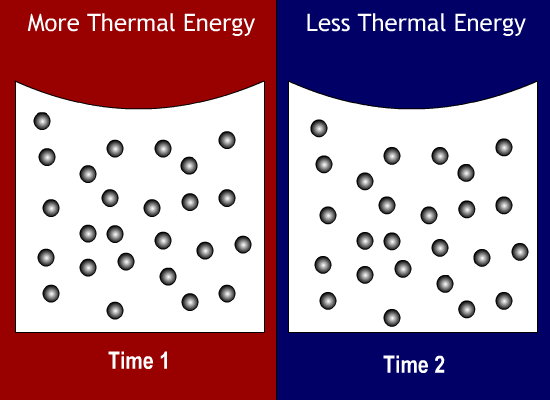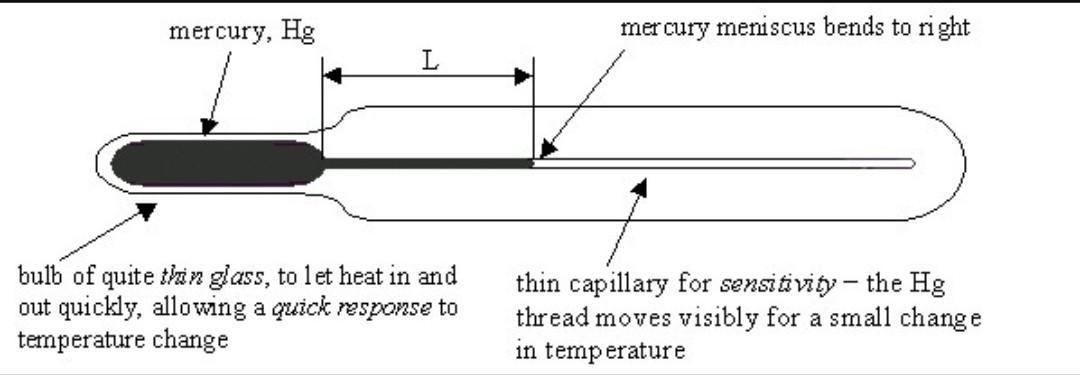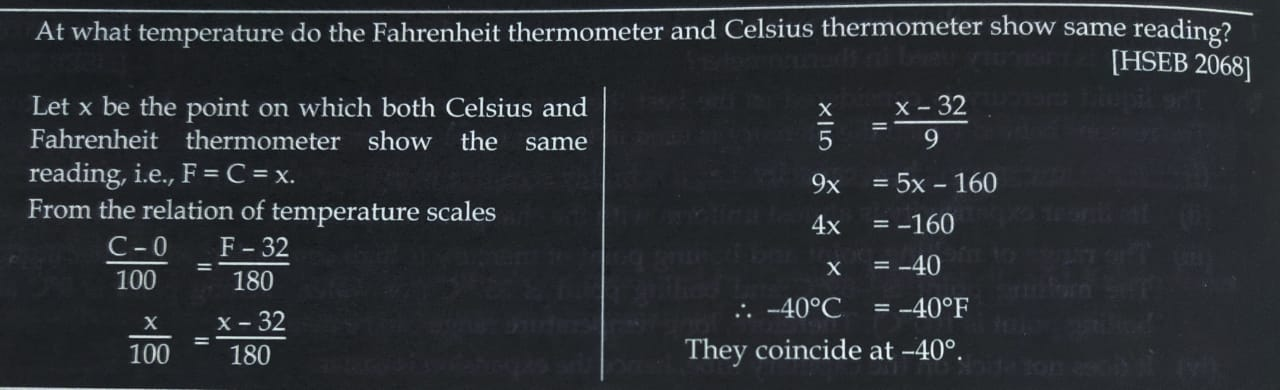# 1.What Is Thermal Energy?

Thermal energy is the energy possessed by an object or system due to the movement of particles within the object or the system. Therefore, thermal energy can be described as the ability of something to do work due to the movement of its particles. So, the faster the particles move within an object or system, the higher the temperature that is recorded.

See following illustration (do not copy in your note)# 2. Heat and Temperature:

Heat and temperature are interconnected, but they do not mean the same thing. If you add heat to something, its temperature goes up. If you reduce temperature, you are taking its heat away.

Knowing the distinction between heat and temperature can lead to a clearer understanding of the world around us.

So, Heat ≠ Temperature

## Why do they differ from one another?

Heat is the total energy of the motion of the molecules of a substance, whereas the temperature refers to the measure of the average energy of the motions of the molecules in the substance. The heat is dependent on factors like the speed of the particles, the size of the particles and the number of particles, etc. The temperature, on the other hand, is independent of these factors. The SI unit of temperature is Kelvin. Temperature is a number that relates to the energy possessed by the molecules of a substance, which directly relates to the kinetic energy possessed by the particles of the substance.# 3. Cause and direction of heat flow:

Heat always flows from high temperature to cold temperature. Simply, hot atoms/molecules vibrate more than cold ones. So a hot vibrating atom hits a cold one and makes the cold one vibrate more. Heat goes from hot to cold because you can’t increase the energy of something without adding energy. The energy comes from the hot side.

What creates a flow of energy is basically the difference in energy between two mediums (regions). What determines the direction of that flow is a universal tendency for systems to move from a state where energy is higher to another where it is lower, in order to reach a state of equilibrium.

A system with certain measurable energy having lower energy neighbouring medium(s), will see its energy flow to said surrounding medium(s) since it will tend towards reaching an equilibrium state where there is no difference in energy between those mediums.

Differences in temperature maintain the transfer of heat, or heat transfer, throughout the universe. Heat transfer is the movement of energy from one place or material to another as a result of a difference in temperature. (You will learn more about heat transfer later in this chapter.)

# 4. Thermal Equilibrium

Temperature measures how hot or cold a body is with respect to a standard object. To discuss temperature changes, two basic concepts are important: thermal contact and thermal equilibrium. Two objects are in thermal contact if they can affect each other’s temperature. Thermal equilibrium exists when two objects in thermal contact no longer affect each other’s temperature.

For example, if a carton of milk from the refrigerator is set on the kitchen countertop, the two objects are in thermal contact. After several hours, their temperatures are the same, and they are then in thermal equilibrium.

# 5. Zeroth Law

The zeroth law is a consequence of thermal equilibrium and allows us to conclude that temperature is a well-defined physical quantity.

The zeroth law of thermodynamics states:

“If a body A and a body B are both in equilibrium with each other; then a body C which is in thermal equilibrium with body B will also be in equilibrium with body and the temperature of body C is equal to the temperature of body A.”

Experimentally, if object A is in equilibrium with object B, and object B is in equilibrium with object C, then object A is in equilibrium with object C. That statement of transitivity is called the zeroth law of thermodynamics. (The number “zeroth” was suggested by British physicist Ralph Fowler in the 1930s).6. Thermal equilibrium as a working principle of a mercury thermometer

A device used to measure the temperature of gaseous, liquid or solid matter or of a chemical reaction such as a fire. All thermometers work on the principle that warm fluids occupy more space than cold fluids. Since we know how much space a fluid such as mercury will fill at any given temperature, we are therefore able to determine the temperature of that fluid by measuring how much space it takes up. The total internal volume of the thermometer tube is precisely engineered, and the thermometer casing carries a series of markers – which indicate the temperature of the liquid to the person using the apparatus.A thermometer measures its own temperature. It is through the concepts of thermal equilibrium and the zeroth law of thermodynamics that we can say that a thermometer measures the temperature of something else, and to make sense of the statement that two objects are at the same temperature. Mercury is the only metal that is a liquid at room temperature, and it is also a poor conductor of heat. The expansion characteristics of mercury are relatively linear compared with many other liquids, meaning that it is possible for it to provide accurate temperature readings. Mercury has a high boiling point, due to its high density. This makes it suitable for taking readings at high temperatures, which makes mercury thermometers especially useful in laboratory settings.

# Temperature scales: Celsius, Fahrenheit and KelvinTemperature scales:

• Celsius, also known as centigrade, is a scale to measure temperature. The unit of measurement is the degree Celsius (°C). It is one of the most commonly used temperature units in the world. The unit system is named after the Swedish astronomer Anders Celsius (1701-1744), who developed a similar temperature scale.On this scale, water’s freezing point is defined to be 0 °C, while water’s boiling point is defined to be 100 °C.
• Fahrenheit scale is based on a scale proposed in 1724 by physicist Daniel Gabriel Fahrenheit (1686-1736). The unit of this scale is the degree Fahrenheit (°F). On this scale, water’s freezing point is defined to be 32 degrees, while water’s boiling point is defined to be 212 degrees.
• Kelvin is a unit of measurement for temperature; the null point of the Kelvin scale is absolute zero, the lowest possible temperature. The Kelvin scale is used extensively in scientific work because a number of physical quantities, such as the volume of an ideal gas, are directly related to absolute temperature.
• The Kelvin is defined as the fraction 1/273.16 of the thermodynamic temperature of the triple point of water (exactly 0.01°C, or 32.018°F).

The relation between three temperature scales:Numerical examples:Define the following terms: Absolute zero, triple point.

• Absolute zero: The coldest possible temperature: zero on the Kelvin scale and approximately -273.15°C and -459.67°F. The total absence of heat; the temperature at which motion of all molecules would cease.

• Triple pointIn thermodynamics, the triple point of a substance is the temperature and pressure at which the three phases of that substance coexist in thermodynamic equilibrium. It is that temperature and pressure at which the sublimation curve, fusion curve, and the vaporization curve meet. For example, the triple point of water and carbon dioxide.For water                                                                        For Carbon dioxide

• Ideal gas:

An ideal gas is a theoretical gas composed of many randomly moving point particles that are not subject to interparticle interactions. The ideal gas concept is useful because it obeys the ideal gas law, a simplified equation of state, and is amenable to analysis under statistical mechanics.